# Consider the following statements. (i) Spring/mass systems and Series Circuit systems we covered are examples of...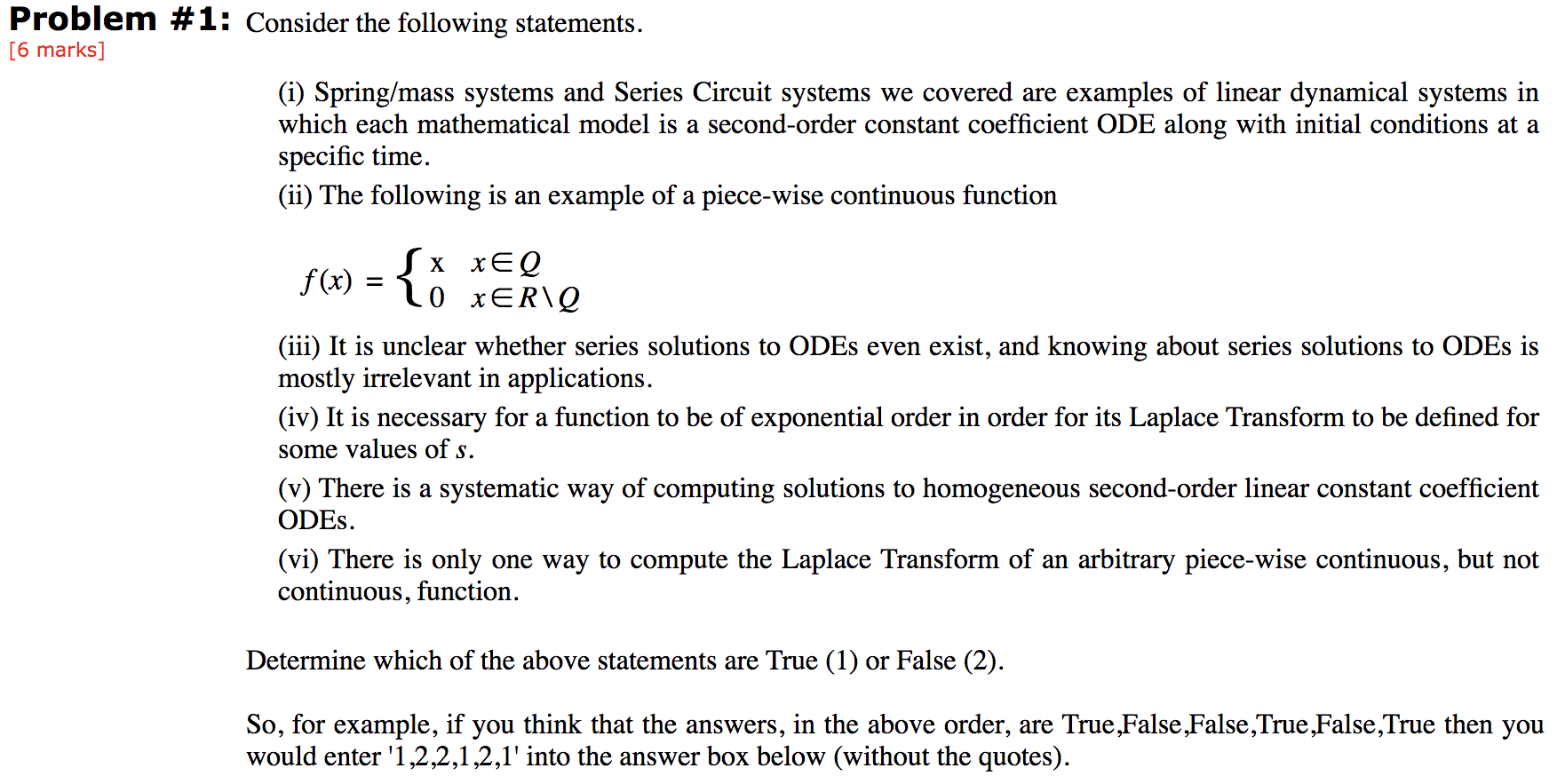Consider the following statements.
(i) Spring/mass systems and Series Circuit systems we covered are examples of linear dynamical systems in which each mathematical model is a second-order constant coefficient ODE along with initial conditions at a specific time.
(ii) The following is an example of a piece-wise continuous function

f (x)  =
 { x x ∈ Q 0 x ∈ R \ Q
(iii) It is unclear whether series solutions to ODEs even exist, and knowing about series solutions to ODEs is mostly irrelevant in applications.
(iv) It is necessary for a function to be of exponential order in order for its Laplace Transform to be defined for some values of s.
(v) There is a systematic way of computing solutions to homogeneous second-order linear constant coefficient ODEs.
(vi) There is only one way to compute the Laplace Transform of an arbitrary piece-wise continuous, but not continuous, function.

Determine which of the above statements are True (1) or False (2).

So, for example, if you think that the answers, in the above order, are True,False,False,True,False,True then you would enter '1,2,2,1,2,1' into the answer box below (without the quotes).
Problem #1: Consider the following statements. [6 marks] (i) Spring/mass systems and Series Circuit systems we covered are examples of linear dynamical systems in which each mathematical model is a second-order constant coefficient ODE along with initial conditions at a specific time. (ii) The following is an example of a piece-wise continuous function f(x) = {* terie (iii) It is unclear whether series solutions to ODEs even exist, and knowing about series solutions to ODEs is mostly irrelevant in applications. (iv) It is necessary for a function to be of exponential order in order for its Laplace Transform to be defined for some values of s. (v) There is a systematic way of computing solutions to homogeneous second-order linear constant coefficient ODES. (vi) There is only one way to compute the Laplace Transform of an arbitrary piece-wise continuous, but not continuous, function. Determine which of the above statements are True (1) or False (2). So, for example, if you think that the answers, in the above order, are True False,False,True False,True then you would enter '1,2,2,1,2,1' into the answer box below (without the quotes).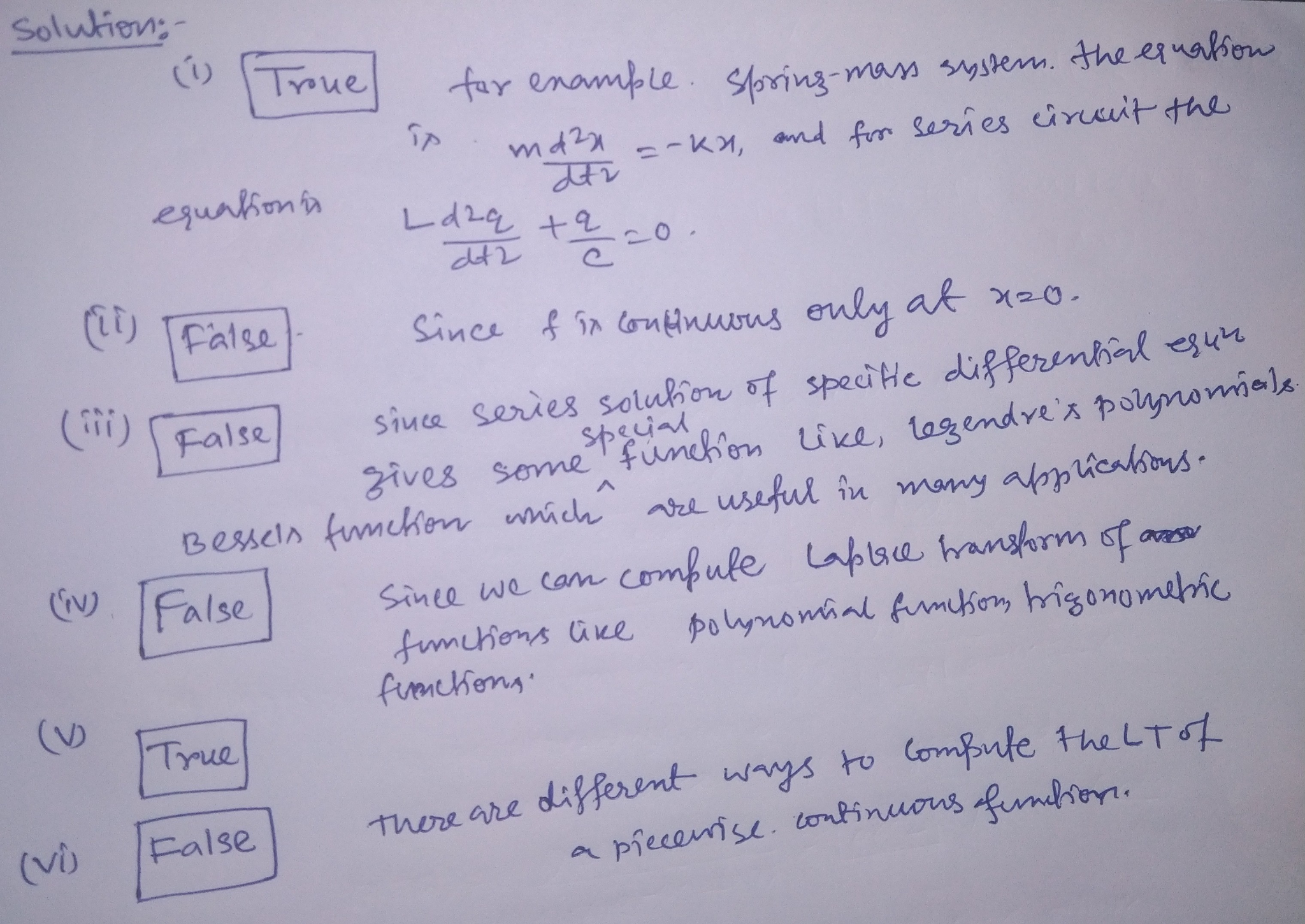##### Add Answer of: Consider the following statements. (i) Spring/mass systems and Series Circuit systems we covered are examples of...
Similar Homework Help Questions
• ### Problem #1: Consider the following statements, [6 marks) 6) There is a systematic way of computing...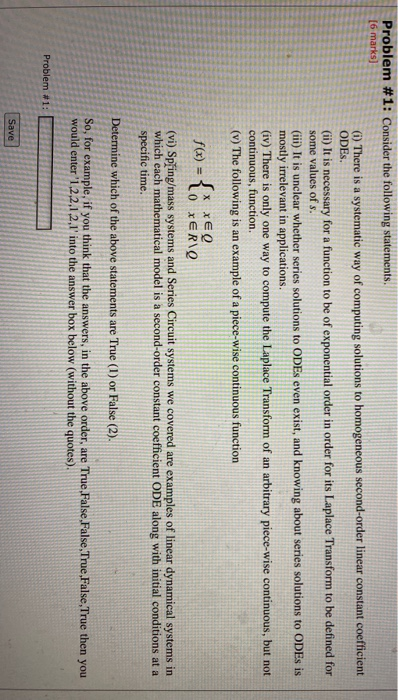Problem #1: Consider the following statements, [6 marks) 6) There is a systematic way of computing solutions to homogeneous second-order linear constant coefficient ODES. (ii) It is necessary for a function to be of exponential order in order for its Laplace Transform to be defined for some values of s. (iii) It is unclear whether series solutions to ODEs even exist, and knowing about series solutions to ODEs is mostly irrelevant in applications. (iv) There is only one way to...

• ### Consider the following statements. (i) The Laplace Transform of 11tet2 cos(et2) is well-defined for some values...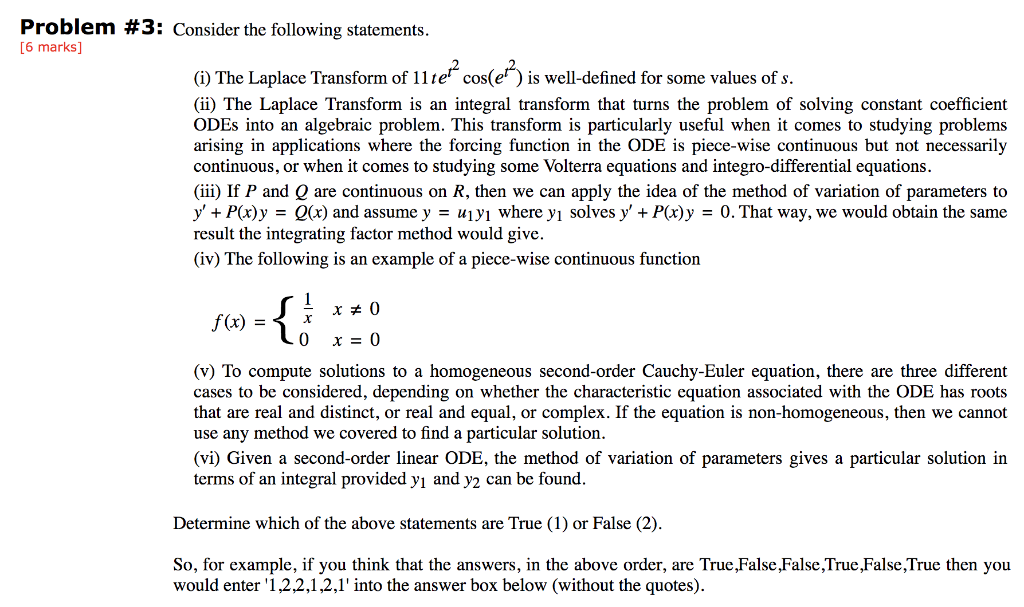Consider the following statements. (i) The Laplace Transform of 11tet2 cos(et2) is well-defined for some values of s. (ii) The Laplace Transform is an integral transform that turns the problem of solving constant coefficient ODEs into an algebraic problem. This transform is particularly useful when it comes to studying problems arising in applications where the forcing function in the ODE is piece-wise continuous but not necessarily continuous, or when it comes to studying some Volterra equations and integro-differential equations. (iii)...

• ### Consider the following statements. (i) Given a second-order linear ODE, the method of variation of parameters...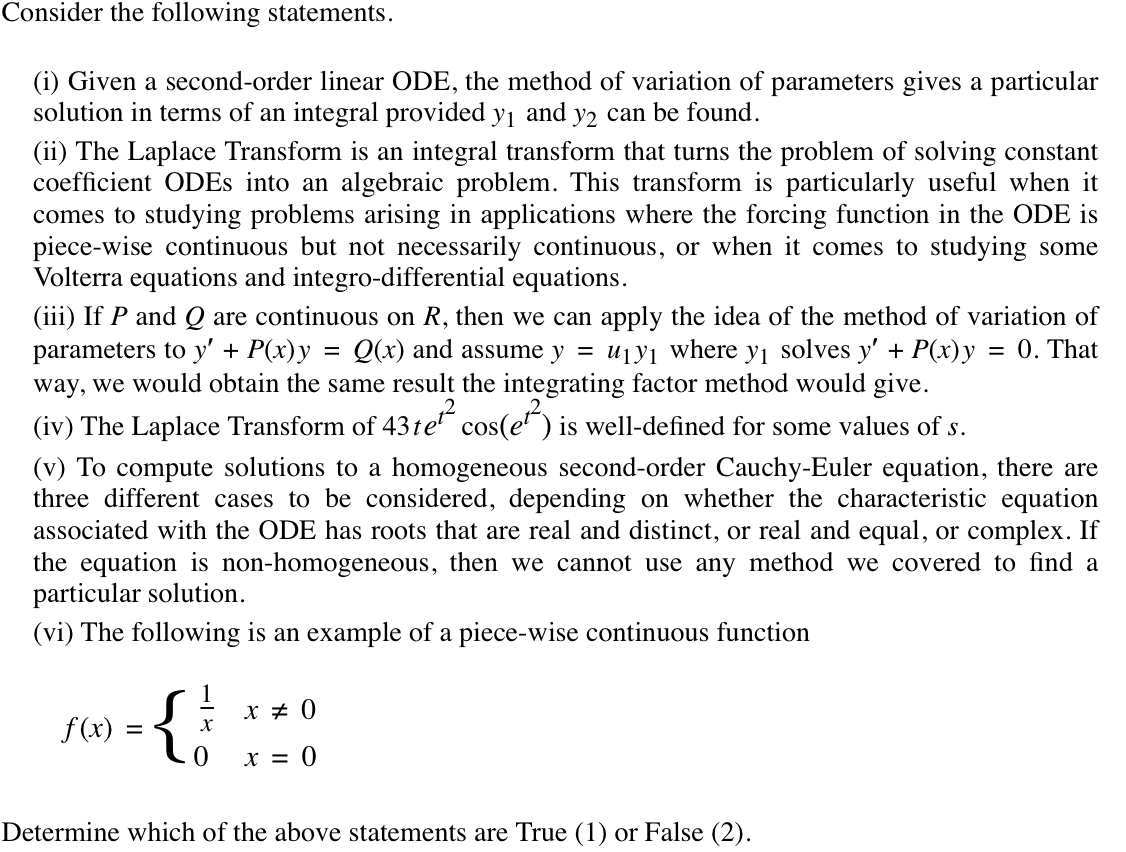Consider the following statements. (i) Given a second-order linear ODE, the method of variation of parameters gives a particular solution in terms of an integral provided y1 and y2 can be found. (ii) The Laplace Transform is an integral transform that turns the problem of solving constant coefficient ODEs into an algebraic problem. This transform is particularly useful when it comes to studying problems arising in applications where the forcing function in the ODE is piece-wise continuous but not necessarily...

• ### Problem #2: Consider the following statements. [6 marks) (1) The particular solution of the ODE)" -...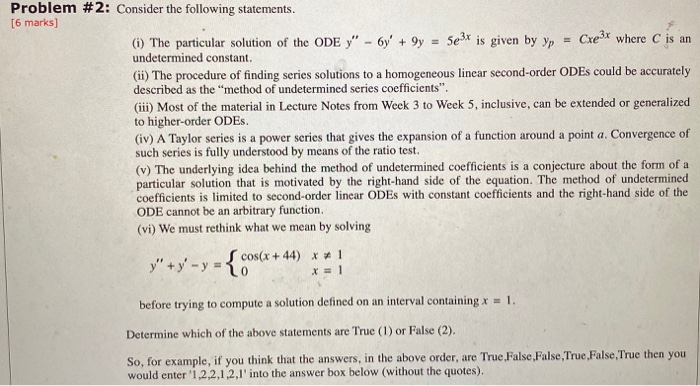Problem #2: Consider the following statements. [6 marks) (1) The particular solution of the ODE)" - 6y' + 9y = 5e3x is given by yp = Cre3x where C is an undetermined constant. (ii) The procedure of finding series solutions to a homogeneous linear second-order ODEs could be accurately described as the "method of undetermined series coefficients". (iii) Most of the material in Lecture Notes from Week 3 to Week 5, inclusive, can be extended or generalized to higher-order ODES...

• ### IGNORE (i) (ii) The procedure of finding series solutions to a homogeneous linear second-order ODEs could...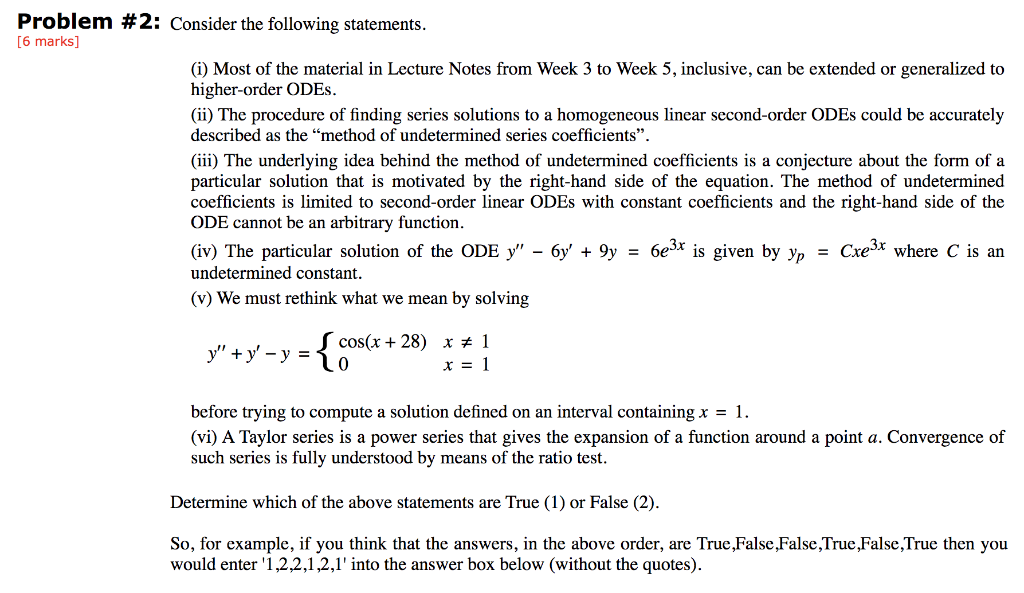IGNORE (i) (ii) The procedure of finding series solutions to a homogeneous linear second-order ODEs could be accurately described as the “method of undetermined series coefficients”. (iii) The underlying idea behind the method of undetermined coefficients is a conjecture about the form of a particular solution that is motivated by the right-hand side of the equation. The method of undetermined coefficients is limited to second-order linear ODEs with constant coefficients and the right-hand side of the ODE cannot be an...

• ### Consider the following statements. (i) A Taylor series is a power series that gives the expansion...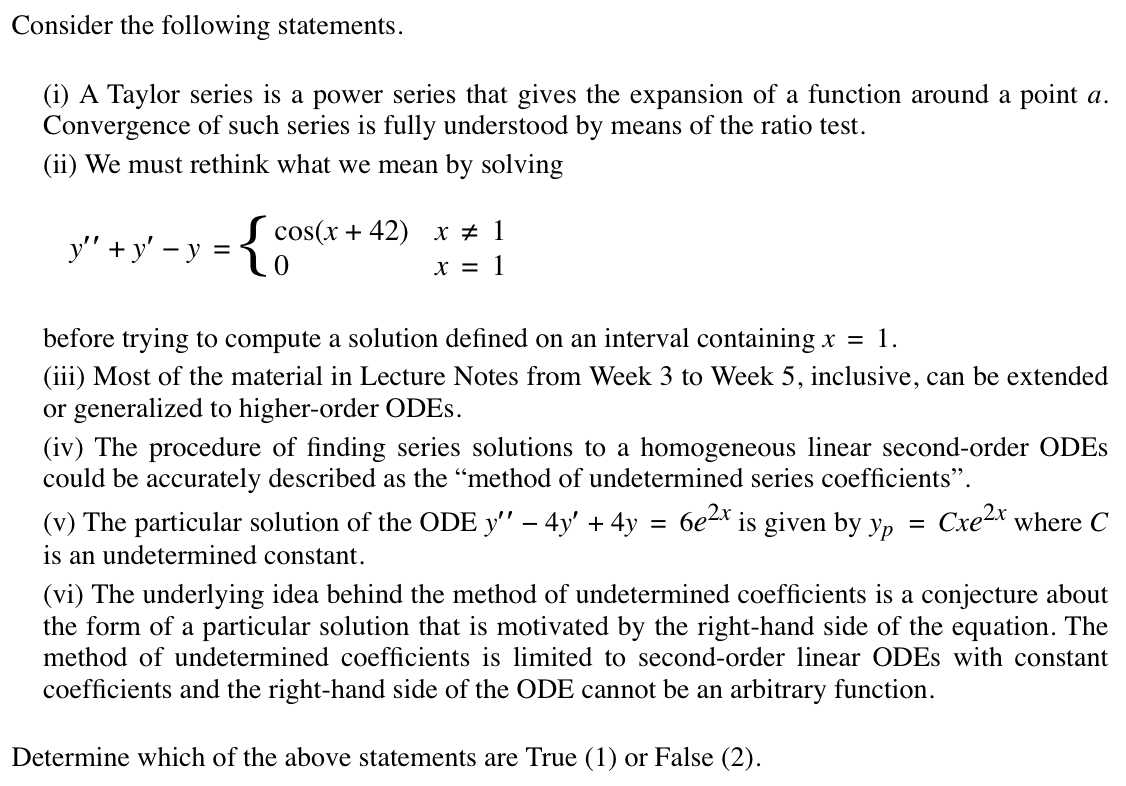Consider the following statements. (i) A Taylor series is a power series that gives the expansion of a function around a point a. Convergence of such series is fully understood by means of the ratio test. (ii) We must rethink what we mean by solving y′′ + y′ − y  =  { cos(x + 42) x  ≠  1 0 x  =  1 before trying to compute a solution defined on an interval containing x  =  1. (iii) Most of the...

• ### 3. State whether the following statements are true or false? You don't need to explain your...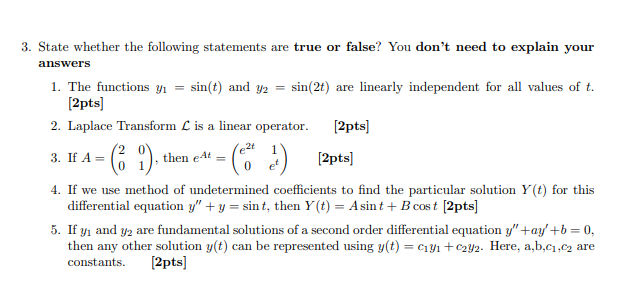3. State whether the following statements are true or false? You don't need to explain your answers 1. The functions yı = sin(t) and y2 = sin(21) are linearly independent for all values of t. [2pts) 2. Laplace Transform C is a linear operator. [2pts) e24 3. If A = then et [2pts) = ( 1), ti 4. If we use method of undetermined coefficients to find the particular solution Y (t) for this differential equation y"+y=sint, then Y (t)...

• ### 1. Consider the following statements:       I. Lapping involves covering a theft related to accounts receivable...

1. Consider the following statements:       I. Lapping involves covering a theft related to accounts receivable by using a later cash receipt to          cover the previous theft.      II. A “positive confirmation” requests that customers respond whether they agree or not with           the amount due to the entity stated in the confirmation.       a. I is true;   II is true       b. I is true;   II is false       c. I is false; II is true       d....

• ### pleas help me with the outline, just need a topic sentence, examples,negative effects or...

DEBATE IN THEPOPULAR PRESS regarding theissue of cell phone useon American roadways is extremely prevalent, highly vituperative and often devoid ofhard data.Editorials are rife with emotional personal accounts oftragedies or near-- tragedies, but suspiciously empty offacts regarding theissues involved or experimentalevidence supporting thepositions espoused.Theresulting public consensus is that in-vehicle use ofthese products presents a danger that must be addressed. Therash oflegislation proposing various types ofquickfixes is proof that lawmakers are being crowded into a position that "something must be done...

• ### How can we assess whether a project is a success or a failure? This case presents...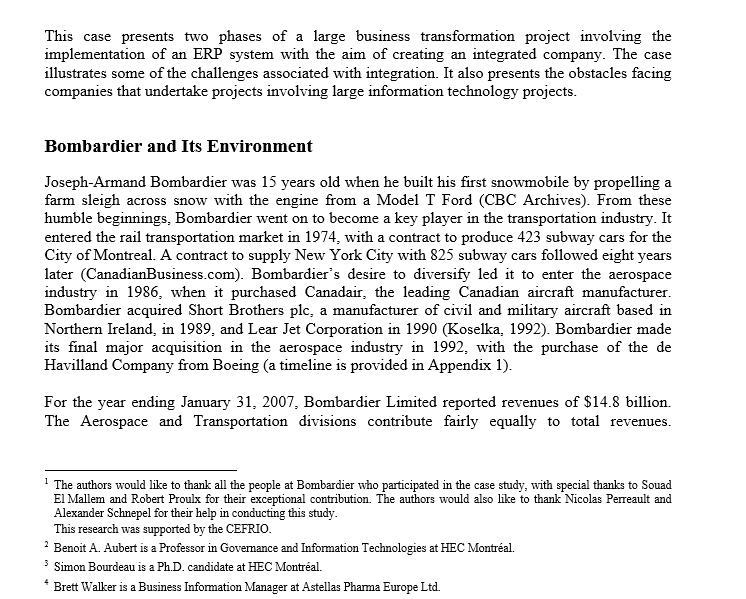How can we assess whether a project is a success or a failure? This case presents two phases of a large business transformation project involving the implementation of an ERP system with the aim of creating an integrated company. The case illustrates some of the challenges associated with integration. It also presents the obstacles facing companies that undertake projects involving large information technology projects. Bombardier and Its Environment Joseph-Armand Bombardier was 15 years old when he built his first snowmobile...

Free Homework App